Question

You have a stopped pipe of adjustable length close to a taut 85.0cm, 7.25g wire under a tension of 4170N. You want to adjust the length of the pipe so that, when it produces sound at its fundamental frequency , this sound causes the wire to vibrate in its second overtone (third harmonic). With a very large amplitude. How long should the pipe be?

1.thanhha

The length is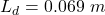Explanation:

From the question we are told that

The length of the wire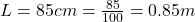The mass is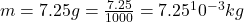The tension is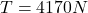Generally the frequency of  oscillation of a stretched wire is mathematically represented as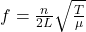Where n is the the number of nodes = 3 (i.e the third harmonic)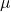is the linear mass density of the wire

This linear mass density is mathematically represented as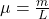Substituting values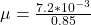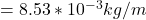Substituting values in to the equation for frequency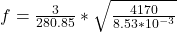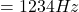From the question the we can deduce that the fundamental frequency is equal to the oscillation of a stretched wire

The fundamental frequency is mathematically represented as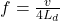Where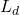is the  length of the pipe

v is the speed of sound with a value of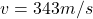Makingthe subject of the formula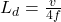Substituting values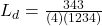From the question the we can deduce that the fundamental frequency is equal to the oscillation of a stretched wire